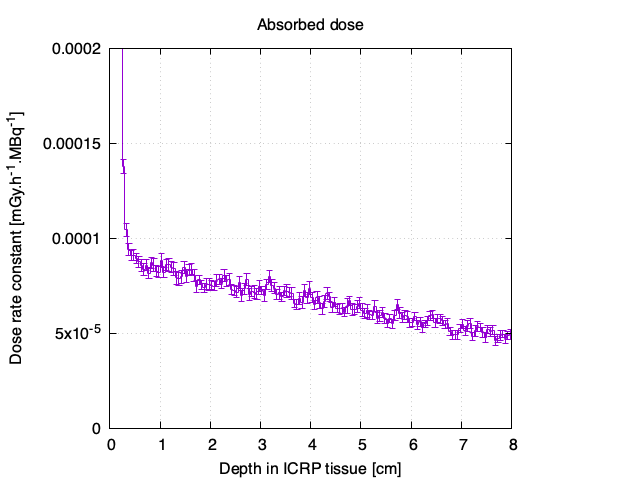# Incorrect value of specific gamma ray constant for 137Cs

I am doing a simple problem to calculate the specific gamma ray constant for 137Cs . I am using isotropic point source at 1 m and scoring dose using userbin card defined using region option.
Now output is 3.4105E-8 by dividing it by volume of 3 inch by 3 inch NAI detector (i.e. 347.5 cm3) and 1.602E-7 GeV/g to Gy conversion factor I got 1.5724E-17 Gy.

By multipliying it with MBq activity and 3600sec for hr I got 0.5661E-4 mSv hr-1 MBq-1.

BUT the gamma-ray constant for Cs-137 given in The Handbook of Health Physics and Radiological Health-Third Edition (p 6-11), 1998 is 1.032 E-04 mSv/h per MBq at 1 meter.

Can anyone help me to find mistake in input or in calculation.

NAI.flair (2.9 KB)
NAI.inp (2.7 KB)

In short: You are comparing Absorbed Dose averaged over your non-negligible detector volume to Ambient Dose Equivalent at a point, which is not the same.

While your simulation is correctly set up (except a small difference in the volume for region NAI, which is rather 319.2 cm3 than 347.5 cm3, according to your input file), the interpretation of what your are calculating and comparing is a bit different.

The dose rate constant \Gamma is usually given for ambient dose equivalent. H*(10) is defined in 10 mm depth in the ICRU sphere, made of ICRU 4-element tissue of density 1 g/cm3. A common value for \Gamma(H*(10)) for Cs-137 is 0.98E-4 mSv.m2.h-1.MBq-1 (JEFF-3.1), so very close to the value you found of 1.032E-04 mSv.m2.h-1.MBq-1.

You may calculate this dose rate constant in two ways with FLUKA:

1. You score photon fluence, at 1 m distance in vacuum, and fold this with fluence-to-dose conversion coefficients for the respective dosimetric quantity. This can be done in one go in FLUKA using DOSE-EQ with the conversion coefficients set AMB74 (AUXSCORE card). The dose rate constant I find is approximately 0.924e-04 mSv.m2.h-1.MBq-1, which is pretty close to the tabulated value.

2. If you use DOSE (Absorbed dose), you have to follow exactly the definition of the dosimetric quantity for H*(10). If you score absorbed dose in your detector this may not be the same as in the tissue equivalent material. In addition you are averaging over a large volume of your detector, while the ambient dose equivalent is defined in a depth of 1 cm.

On this graph you see the evolution of the dose rate constant as a function of depth in your detector where I had replaced all materials with ICRP 4-element tissue (for simplicity, I did not follow the exact definition for H*(10) for geometry and radiation field). You see that at 1 cm depth, the value is approximately 0.9E-4 mGy.h-1.MBq-1, so it is pretty close to the tabulated dose rate constant for H*(10).In this graph you see the same, but for your detector with the original materials. You can see that a value averaged over the full volume of the detector will deviate from the tabulated dose rate constant they way you have observed.I hope that clarifies your observation/question.

Kind regards,

Markus

2 Likes

Thank you Sir for full detailed response.